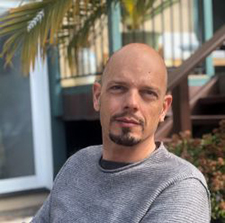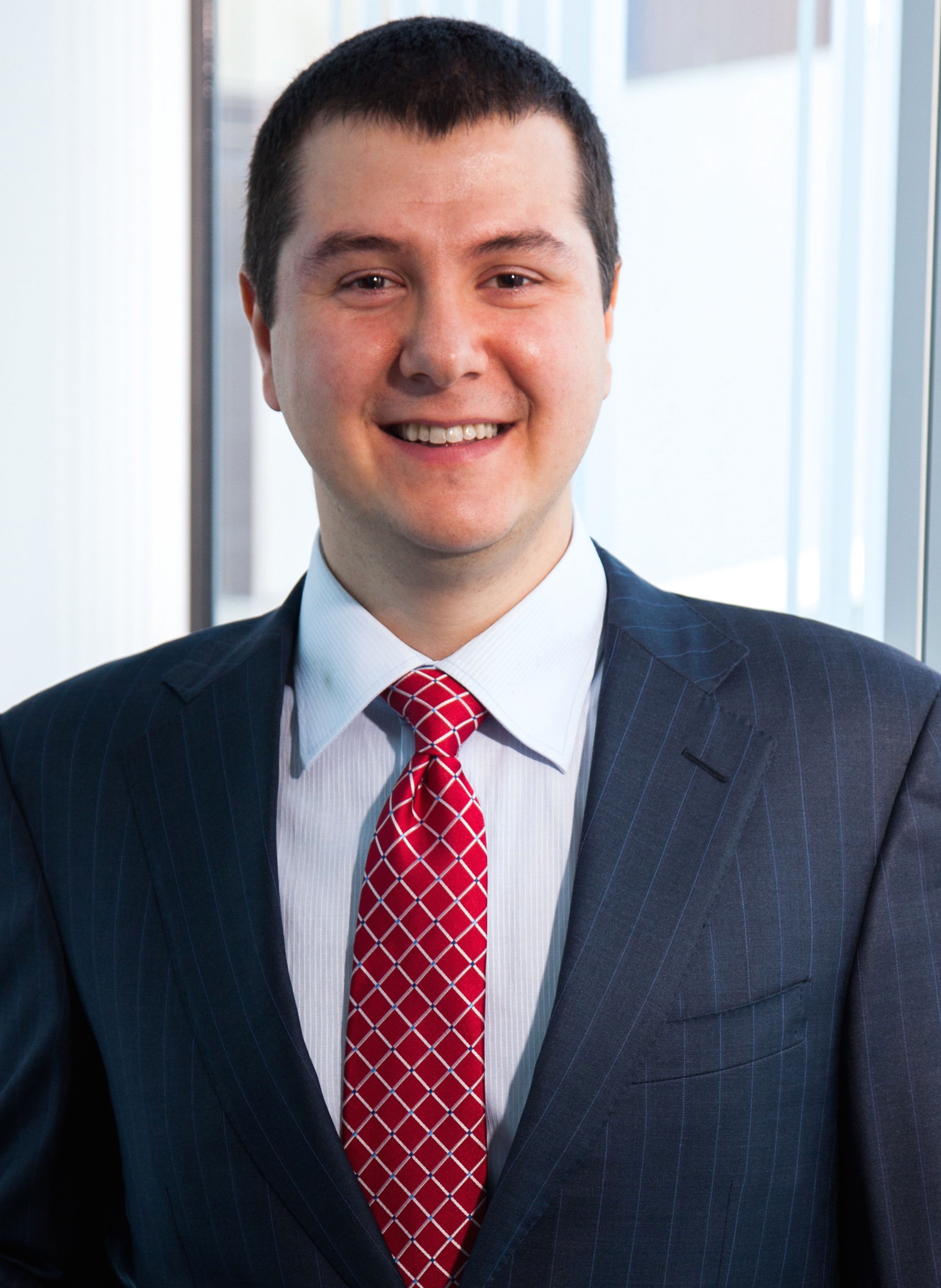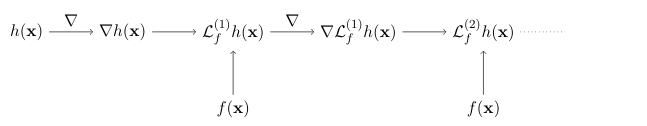## Probabilistic Safety Constraints

### for Learned High Relative Degree System DynamicsMohammad Javad Khojasteh$$^*$$Vikas Dhiman$$^*$$Massimo FranceschettiNikolay Atanasov $$^*$$ These authors contributed equally.

## Today's focus##### Given:
• Map and localization (Full observability)
• Desired trajectory as a plan
• Unsafe regions
##### Unknown:
• Robot system dynamics
##### Want:
• Follow trajectory avoiding unsafe actions

## Problem formulation• \begin{align} \label{eq:system_dyanmics} \dot{\bfx} = f(\bfx) + g(\bfx)\bfu = \begin{bmatrix} f(\bfx) & g(\bfx)\end{bmatrix} \begin{bmatrix}1\\\bfu\end{bmatrix} =: F(\bfx) \ctrlaff \end{align}
• $\vect(F(\bfx)) \sim \GP(\vect(\bfM_0(.)), \bfK_0(.,.))$
• \begin{align} \min_{\bfu_k \in \mathcal{U}}& \text{ Task cost function } \\ \qquad\text{s.t.}&~~\bbP\bigl( \text{ Safety constraint } \mid \bfx_k,\bfu_k \bigr) \ge \tilde{p}_k, \end{align}
\begin{align} \min_{\bfu_k \in \mathcal{U}}& \|\bfu_k - \cssId{highlight-border-red-1}{\class{fragment}{\pi_\epsilon(\bfx_k)}} \|_Q \\ \qquad\text{s.t.}&~~\bbP\bigl( \cssId{highlight-border-red-1}{\class{fragment}{h(\bfx) > \zeta_h > 0}} \mid \bfx_k,\bfu_k \bigr) \ge \tilde{p}_k, \end{align}

## Approach

• Estimate $$F(\bfx)$$ with uncertainity.
• Propagate uncertainty to the Safety condition.
• Extension to continous time using Lipchitz continuity assumptions.
• Extension to higher relative degree systems.

## Matrix Variate Gaussian Processes

$\vect(F(\bfx)) \sim \GP(\vect(\bfM_0(.)), \bfK_0(.,.))$
Option 1: Learn each matrix element independently $\bfK_0(\bfx, \bfx')_{i, j} = \kappa(\bfx, \bfx')$ No correlation across dimensions
Option 2: Alvarez et al (FTML 2012): $\bfK_0(\bfx, \bfx') = \kappa(\bfx, \bfx') \boldsymbol{\Sigma}$ $$\Sigma \in \R^{n(1+m) \times (1+m)n}$$ has too many parameters to learn
Option 3: Sun et al (AISTATS 2017)
$F \sim \mathcal{MVG}(\bfM, \bfA, \bfB) \Leftrightarrow \vect(F) \sim \calN(\vect(M), \bfB \otimes \bfA)$
$\bfK_0(\bfx, \bfx') = \bfB_0(\bfx, \bfx') \otimes \bfA$

Factorization assumption: $\vect(F(\bfx)) \sim \GP(\vect(\bfM_0(.)), \bfB_0(.,.) \otimes \bfA)$

## Matrix variate Gaussian Process

$$\newcommand{\prl}{\left(#1\right)} \newcommand{\brl}{\left[#1\right]} \newcommand{\crl}{\left\{#1\right\}}$$ \begin{equation} \begin{aligned} \vect(F(\bfx)) &\sim \mathcal{GP}(\vect(\bfM_0(\bfx)), \bfB_0(\bfx,\bfx') \otimes \bfA) %F(\bfx)\underline{\bfu} &\sim \mathcal{GP}(\bfM_0(\bfx)\underline{\bfu}, \underline{\bfu}^\top \bfB_0(\bfx,\bfx') \underline{\bfu}' \otimes \bfA) \end{aligned} \end{equation}
Given data $$\StDat_{1:k} := [\bfx(t_1), \dots, \bfx(t_k)]$$, $$\StDtDat_{1:k}=[\dot{\bfx}(t_1), \dots, \dot{\bfx}(t_k)]$$, and $$\underline{\boldsymbol{\mathcal{U}}}_{1:k}:= \diag(\ctrlaff_1, \dots, \ctrlaff_k)$$.
\begin{equation*} \begin{aligned} \bfM_k(\bfx_*) &:= \bfM_0(\bfx_*) + \prl{ \dot{\bfX}_{1:k} - \boldsymbol{\mathcal{M}}_{1:k}\underline{\boldsymbol{\mathcal{U}}}_{1:k}} \prl{\underline{\boldsymbol{\mathcal{U}}}_{1:k}^\top\bfB_0(\bfX_{1:k},\bfX_{1:k})\underline{\boldsymbol{\mathcal{U}}}_{1:k}}^{-1}\underline{\boldsymbol{\mathcal{U}}}_{1:k}^\top\bfB_0(\bfX_{1:k},\bfx_*)\\ \bfB_k(\bfx_*,\bfx_*') &:= \bfB_0(\bfx_*,\bfx_*') + \bfB_0(\bfx_*,\bfX_{1:k})\underline{\boldsymbol{\mathcal{U}}}_{1:k}\prl{\underline{\boldsymbol{\mathcal{U}}}_{1:k}^\top\bfB_0(\bfX_{1:k},\bfX_{1:k})\underline{\boldsymbol{\mathcal{U}}}_{1:k}}^{-1}\underline{\boldsymbol{\mathcal{U}}}_{1:k}^\top\bfB_0(\bfX_{1:k},\bfx_*') \label{eq:mvg-posterior} \end{aligned} \end{equation*}
Inference on MVGP: \begin{align} \vect(F_k(\bfx_*)) &\sim \mathcal{GP}(\vect(\bfM_k(\bfx_*)), \; \bfB_k(\bfx_*,\bfx_*') \otimes \bfA). \\ F_k(\bfx_*)\underline{\bfu}_* &\sim \mathcal{GP}(\bfM_k(\bfx_*)\underline{\bfu}_*, \; \underline{\bfu}_*^\top\bfB_k(\bfx_*,\bfx_*')\underline{\bfu}_*\otimes\bfA). \end{align}

## Approach

• Estimate $$F(\bfx)$$ with Matrix-Variate Gaussian Process
• Propagate uncertainty to the Safety condition
• Extension to continous time using Lipchitz continuity assumptions.
• Extension to higher relative degree systems.

## Control Barrier Functions• For differentiable $$h(\bfx)$$,
safe set is $$\calC = \{ \bfx \in \calX : h(\bfx) > 0 \}$$
• Assume $$\grad_\bfx h(\bfx) \ne 0 \quad \forall x \in \partial \calC$$
• Assume system starts in safe state $$\bfx(0) \in \calC$$
• Ames et al (ECC 2019): \begin{multline} \text{ System stays safe } \Leftrightarrow~~\exists~\bfu = \pi(\bfx)~~\text{s.t.}\\ \mbox{CBC}(\bfx,\bfu) := \Lie_f h(\bfx) + \Lie_g h(\bfx)\bfu + \alpha(h(\bfx)) \ge 0 \;~ \forall \bfx \in \calX. \end{multline} where $$\alpha(y)$$ is some extended class $$\calK_\infty$$ function

## Uncertainity propagation to CBC

• \begin{align} \mbox{CBC}(\bfx, \bfu) &:= \Lie_{f}h(\bfx) + \Lie_{g}h(\bfx)\bfu + \alpha(h(\bfx)) \end{align}
• $\mbox{CBC}(\bfx, \bfu)= \grad_\bfx h(\bfx)F_k(\bfx)\ctrlaff + \alpha(h(\bfx))$
• Recall: \begin{equation} F_k(\bfx_*)\underline{\bfu}_* \sim \mathcal{GP}(\bfM_k(\bfx_*)\underline{\bfu}_*, \underline{\bfu}_*^\top\bfB_k(\bfx_*,\bfx_*')\underline{\bfu}_*\otimes\bfA). \end{equation}
• Lemma : $\mbox{CBC}(\bfx, \bfu) \sim \GP(\E[\mbox{CBC}], \Var(\mbox{CBC}))$ \begin{align} \label{eq:parametofpi5543} \E[\mbox{CBC}_k](\bfx, \bfu) &= \nabla_\bfx h(\bfx)^\top \bfM_k(\bfx)\underline{\bfu} + \alpha(h(\bfx)),\\ \Var[\mbox{CBC}_k](\bfx, \bfx'; \bfu) &= \underline{\bfu}^\top\bfB_k(\bfx,\bfx')\underline{\bfu} \nabla_\bfx h(\bfx)^{\top}\bfA\nabla_\bfx h(\bfx') \end{align} Note: mean and variance are Affine and Quadratic in $$\bfu$$ respectively.

## Deterministic condition for controller

• \begin{align} \min_{\bfu_k \in \mathcal{U}}& \text{ Task cost function } \\ \qquad\text{s.t.}&~~\bbP\bigl( \text{ Safety constraint } \mid \bfx_k,\bfu_k \bigr) \ge \tilde{p}_k, \end{align}
\begin{align} \min_{\bfu_k \in \mathcal{U}}& \|\bfu_k - \pi_\epsilon(\bfx_k) \|_Q \\ \qquad\text{s.t.}&~~\bbP\bigl( \style{color:red}{\mbox{CBC}(\bfx_k, \bfu_k) > \zeta > 0} \mid \bfx_k,\bfu_k \bigr) \ge \tilde{p}_k, \end{align}
• $\newcommand{\CBC}{\mbox{CBC}} \bbP\bigl(\mbox{CBC}(\bfx_k, \bfu_k) > \zeta \mid \bfx_k,\bfu_k \bigr) \ge \tilde{p}_k \\ \Leftrightarrow \frac{1}{2}-\frac{1}{2} \erf\left( \frac{\zeta - \E[\CBC] }{\sqrt{2\Var(\CBC)}} \right) \ge \tilde{p}_k$ where $$\erf(y)$$ is there error function.
• Safe controller (a QCQP): \begin{align} \min_{\bfu_k \in \mathcal{U}}& \|\bfu_k - \pi_\epsilon(\bfx_k) \|_Q \\ \qquad\text{s.t.}\qquad& \cssId{highlight-current-red-1}{\class{fragment}{ (\E[\CBC] - \zeta)^2 \ge 2\Var(\CBC)(\erf^{-1}(1-2\tilde{p}_k))^2 }} \\ & \cssId{highlight-current-red-1}{\class{fragment}{ \E[\CBC] - \zeta \ge 0 }} \end{align}

## Approach

• Estimate $$F(\bfx)$$ with Matrix-Variate Gaussian Process
• Propagate uncertainty to the Control Barrier condition.
• Extension to continous time using Lipchitz continuity assumptions.
• Extension to higher relative degree systems.

## Safety beyond triggering times

• So far: \begin{align} \min_{\bfu_k \in \mathcal{U}}& \|\bfu_k - \pi_\epsilon(\bfx_k) \|_Q \\ \qquad\text{s.t.}&~~ \bbP\bigl( \mbox{CBC}(\style{color:red}{\bfx_k}, \bfu_k) > \style{color:red}{\zeta} \mid \bfx_k,\bfu_k \bigr) \ge \style{color:red}{\tilde{p}_k}, \end{align}
• Next: \begin{align} \min_{\bfu_k \in \mathcal{U}}& \|\bfu_k - \pi_\epsilon(\bfx_k) \|_Q \\ \qquad\text{s.t.}&~~ \bbP\bigl( \mbox{CBC}(\style{color:red}{\bfx(t)}, \bfu_k) > \style{color:red}{0} \mid \bfx_k,\bfu_k \bigr) \ge \style{color:red}{p_k}, \qquad \style{color:red}{\forall t \in [t_k, \tau_k)} \end{align}

## Safety beyond triggering times

• Assume Lipchitz continuity of dynamics: \begin{align} \textstyle \label{eq:smoth23} \bbP\left( \sup_{s \in [0, \tau_k)}\|F(\bfx(t_k+s))\ctrlaff_k -F(\bfx(t_k))\ctrlaff_k\| \le L_k \|\bfx(t_k+s)-\bfx_k\| \right) \ge q_k:=1-e^{-b_kL_k}. \end{align}
• Assume Lipchitz continuity of $$\alpha(h(\bfx))$$: \begin{align} \label{htym6!7uytf} |\alpha \circ h(\bfx(t_k+s))-\alpha \circ h(\bfx_k)| \le L_{\alpha \circ h} \|\bfx(t_k+s)-\bfx_k\|. \end{align}
Theorem: $\bbP\bigl( \mbox{CBC}(\bfx_k, \bfu_k) > \zeta \mid \bfx_k,\bfu_k \bigr) \ge \tilde{p}_k \quad\Rightarrow\quad \bbP\bigl( \mbox{CBC}(\bfx(t), \bfu_k) > 0 \mid \bfx_k,\bfu_k \bigr) \ge p_k, \; \forall t \in [t_k, \tau_k)$ holds with $$p_k = \tilde{p}_k q_k$$ and $$\tau_k \le \frac{1}{L_k}\ln\left(1+\frac{L_k\zeta}{(\chi_kL_k+L_{\alpha \circ h})\|\dot{\bfx}_k\|}\right)$$

## Approach

• Estimate $$F(\bfx)$$ with Matrix-Variate Gaussian Process
• Propagate uncertainty to the Control Barrier condition.
• Extension to continous time using Lipchitz continuity assumptions.
• Extension to higher relative degree systems.

## Higher relative degree CBFs• \begin{align} \begin{bmatrix} \dot{\theta} \\ \dot{\omega} \end{bmatrix} = \underbrace{\begin{bmatrix} \omega \\ -\frac{g}{l} \sin(\theta) \end{bmatrix}}_{f(\bfx)} + \underbrace{\begin{bmatrix} 0 \\ \frac{1}{ml} \end{bmatrix}}_{g(\bfx)} u \end{align}
• \begin{align} h\left(\begin{bmatrix} \theta \\ \omega \end{bmatrix} \right) = \cos(\Delta_{col}) - \cos(\theta - \theta_c) \end{align}
• Note that $$\Lie_g h(\bfx) = \grad h(\bfx) g(\bfx) = 0$$
• Thus $$\CBC(\bfx, \bfu)$$ is independent of u.

## Exponential Control Barrier Functions (ECBF)

• $\CBCr(\bfx, \bfu) := \Lie_f^{(r)} h(\bfx) + \Lie_g \Lie_f^{(r-1)} h(\bfx) \bfu + K_\alpha \begin{bmatrix} h(\bfx) \\ \Lie_f h(\bfx) \\ \vdots \\ \Lie_f^{(r-1)} h(\bfx) \end{bmatrix}$
• $$r \ge 1$$ is the relative degree of CBF, $$h(\bfx)$$, then $$\Lie_g \Lie_f^{k} h(\bfx) = 0, \; \forall k = \{0, \dots, r-2 \}$$ and $$\Lie_g \Lie_f^{(r-1)} h(\bfx) \ne 0$$ and

## Propagating uncertainity to $$\CBCtwo$$

• $\CBCtwo(\bfx, \bfu) = [\grad_\bfx \Lie_f h(\bfx)]^\top F(\bfx)\ctrlaff + K_\alpha \begin{bmatrix} h(\bfx) & \Lie_f h(\bfx) \end{bmatrix}^\top$
• $$\Lie_f h(\bfx) = \grad_x h(\bfx) f(\bfx)$$ is a Gaussian process
• $$\grad_\bfx \Lie_f h(\bfx)$$ is a Gaussian process
• If $$p(\bfx) \sim \GP(\mu(\bfx), \kappa(\bfx, \bfx'))$$, then
$$\grad_\bfx p(\bfx) \sim \GP(\grad_\bfx \mu(\bfx), H_\bfx \kappa(\bfx, \bfx'))$$

## Propagating uncertainity to $$\CBCtwo$$

• $\CBCtwo(\bfx, \bfu) = [\grad_\bfx \Lie_f h(\bfx)]^\top F(\bfx)\ctrlaff + K_\alpha \begin{bmatrix} h(\bfx) & \Lie_f h(\bfx) \end{bmatrix}^\top$
• $$\Lie_f h(\bfx) = \grad_x h(\bfx) f(\bfx)$$ is a Gaussian process
• $$\grad_\bfx \Lie_f h(\bfx)$$ is a Gaussian process
• $$[\grad_\bfx \Lie_f h(\bfx)]^\top F(\bfx)\ctrlaff$$ is a quadratic form of GP (not a GP )
• $$\newcommand{\trc}{\text{tr}}$$ If $$p(\bfx)$$ and $$q(\bfy)$$ are GPs then $$p(\bfx)^\top q(\bfx)$$ is also a GP
\begin{multline} p(\bfx)^\top q(\bfx) \sim \GP(\mu_p(\bfx)^\top \mu_q(\bfx) + \trc(\Cov_{p,q}(\bfx, \bfx)), \\ 2\trc(\Cov_{p,q}(\bfx, \bfx'))^2 ) + p(\bfx)^\top \kappa_q(\bfx, \bfx') p(\bfx') \\ + q(\bfx)^\top \kappa_p(\bfx, \bfx') q(\bfx') + 2 q(\bfx)^\top \Cov_{p,q}(\bfx, \bfx') p(\bfx') \end{multline}
• $$\CBCtwo(\bfx, \bfu)$$ is a quadratic form of GP.
$$\E[\CBCtwo](\bfx, \bfu)$$ is still affine in $$\bfu$$.
$$\Var[\CBCtwo](\bfx, \bfx'; \bfu)$$ is still quadratic in $$\bfu$$.

## Extending to $$\CBCr$$

• $\CBCr(\bfx, \bfu) = [\grad_\bfx \Lie_f^{(r)} h(\bfx)]^\top F(\bfx)\ctrlaff + K_\alpha \begin{bmatrix} h(\bfx) & \Lie_f h(\bfx) & \dots \Lie_f^{(r-1)} h(\bfx) \end{bmatrix}^\top$
•• $$\CBCr(\bfx, \bfu)$$ is not a GP
$$\E[\CBCr](\bfx, \bfu)$$ is still affine in $$\bfu$$.
$$\Var[\CBCr](\bfx, \bfx'; \bfu)$$ is still quadratic in $$\bfu$$.
• For $$r \ge 3$$, $$\CBCr$$ statistics can be estimated by Monte-carlo methods.

## Safe controller using ECBF

• \begin{align} \min_{\bfu_k \in \mathcal{U}}& \|\bfu_k - \pi_\epsilon(\bfx_k) \|_Q \\ \qquad\text{s.t.}&~~ \bbP\bigl( \CBCr(\bfx_k, \bfu_k) > \zeta \mid \bfx_k,\bfu_k \bigr) \ge \tilde{p}_k \end{align}
• Using Cantelli's (Chebyshev's one-sided) inequality
• Safe controller (a QCQP) \begin{align} \min_{\bfu_k \in \mathcal{U}}& \|\bfu_k - \pi_\epsilon(\bfx_k) \|_Q \\ \qquad\text{s.t.}\qquad &(\E[\mbox{CBC}_k^{(r)}]-\zeta)^2 \ge \frac{\tilde{p}_k}{1-\tilde{p}_k}\Var[\mbox{CBC}_k^{(r)}] \\ &\E[\mbox{CBC}_k^{(r)}]-\zeta \ge 0 \end{align}

## Learning Experiments• \begin{align} \begin{bmatrix} \dot{\theta} \\ \dot{\omega} \end{bmatrix} = \underbrace{\begin{bmatrix} \omega \\ -\frac{g}{l} \sin(\theta) \end{bmatrix}}_{f(\bfx)} + \underbrace{\begin{bmatrix} 0 \\ \frac{1}{ml} \end{bmatrix}}_{g(\bfx)} u \end{align}
• \begin{align} h\left(\begin{bmatrix} \theta \\ \omega \end{bmatrix} \right) = \cos(\Delta_{col}) - \cos(\theta - \theta_c) \end{align}## Safe controller using ECBF Experiments• \begin{align} \begin{bmatrix} \dot{\theta} \\ \dot{\omega} \end{bmatrix} = \underbrace{\begin{bmatrix} \omega \\ -\frac{g}{l} \sin(\theta) \end{bmatrix}}_{f(\bfx)} + \underbrace{\begin{bmatrix} 0 \\ \frac{1}{ml} \end{bmatrix}}_{g(\bfx)} u \end{align}
• \begin{align} h\left(\begin{bmatrix} \theta \\ \omega \end{bmatrix} \right) = \cos(\Delta_{col}) - \cos(\theta - \theta_c) \end{align}## Take away

• Safety guarantees in stochastic control-affine systems were formuated as Quadratic contraints on the control signal using Exponential Control Barrier Functions.

## Ongoing work

• More experiments (closer to the Motivation).
• What if QCQP is non-convex?
• Entropy objective to pick optimal actions for reducing uncertainity.
• Application of Hansen-Wright like inequalities for tighter bounds on $$\CBCr$$

# Thank you. Questions?

#### Paper URL: arxiv.org/abs/1912.10116

$$^*$$ These authors contributed equally.